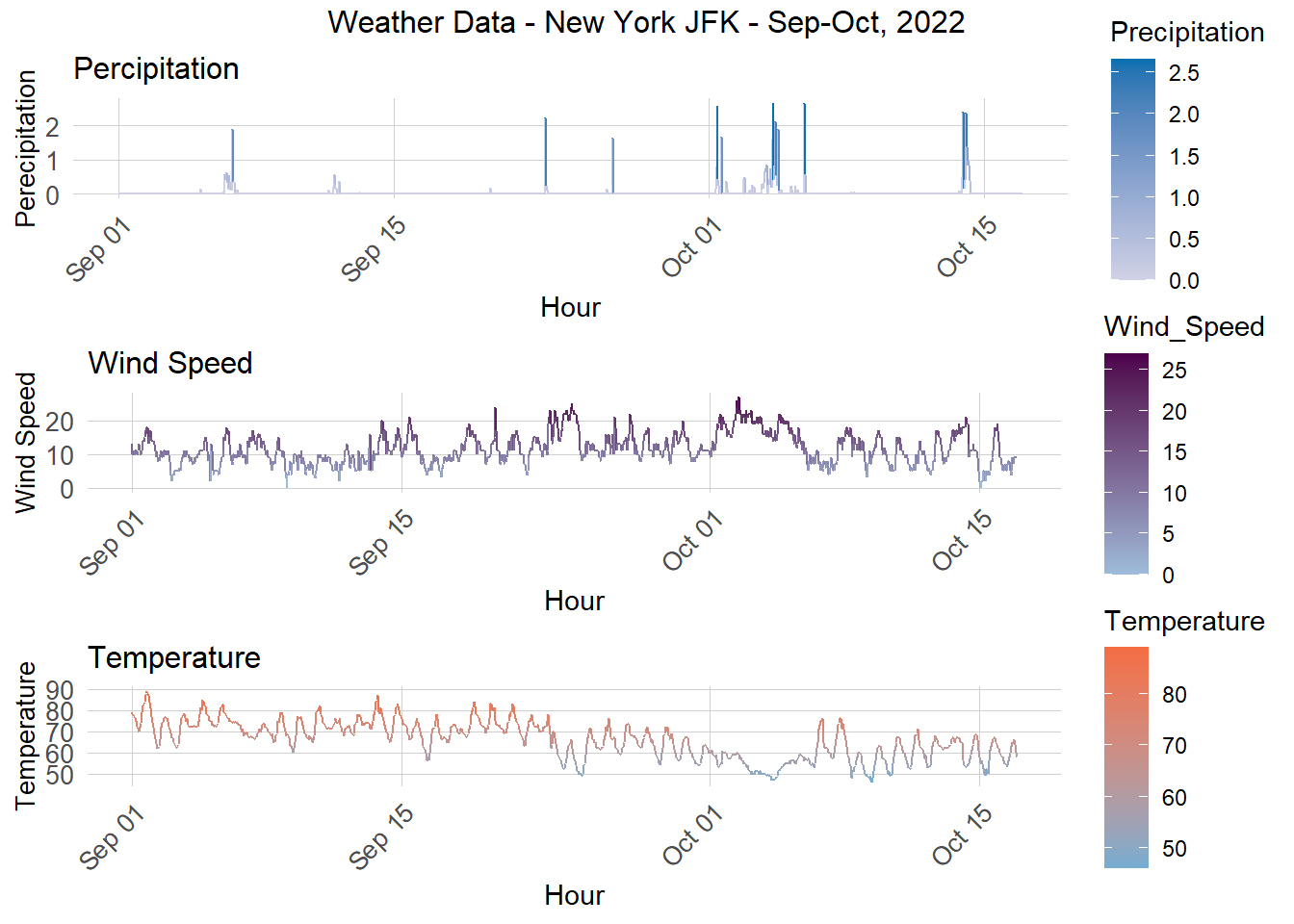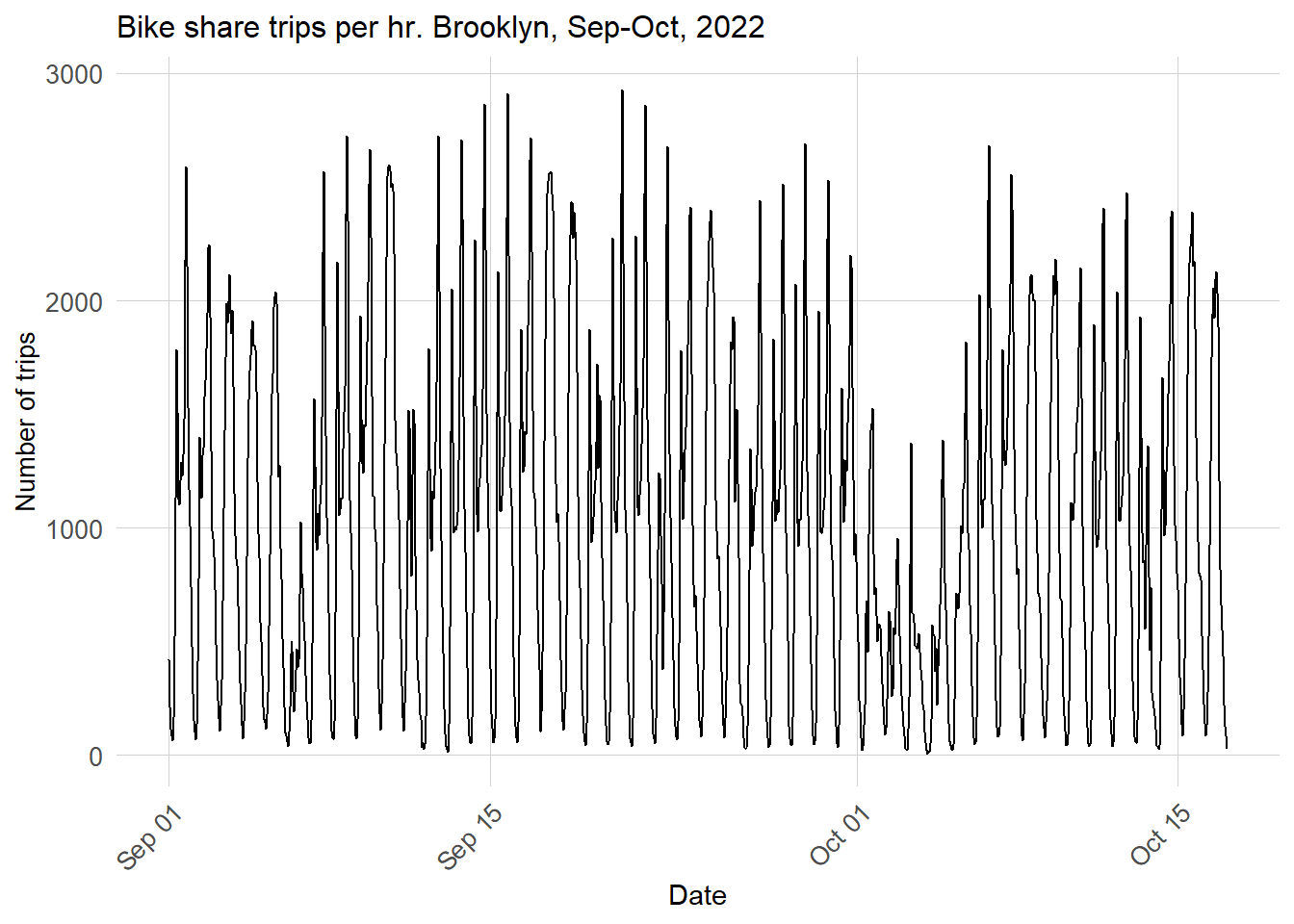# Introduction

Citibike lets New Yorkers hop on a bike when they want, to go wherever they want. No more worrying about where to lock up a bike - Citibike’s stations have dedicated slots to securely return your bikes. And no more having to schlep your bike home - bike share means that you can take one-way trips and not have to worry about your bike when you’re done. It’s convenient, easy, and cheap.

This convenience of on-demand bikes creates a puzzle for Citibike’s logistics: how to ensure that bikes are available where and when they’re needed. If bikes aren’t available at the right place and the right time, ridership and revenue will fall. It’s therefore very important to rebalance bikes in the system: i.e. move bikes from areas of low demand to areas of high demand, before the high demand begins.

To help Citibike rebalance bikes, I’ve created a model to forecast demand in Brooklyn. My rebalancing plan will work as follows:

• Pre-peak incentives: my program would provide incentives for riders to park their bikes in stations that are forecast to have high demand in the one- to two-hour windows before the higher demand occurs. These incentives would include: a \$2* discount per day pass or monthly membership; and a reserved slot at the receiving station that ensure riders will definitely have a place to park - a perk otherwise not available in the system. (*Note, this is an example discount rate. This discount may be adjusted based on further research into cost and benefit thresholds.)

• Overnight trucking: Morning demand is spread across residential areas, making it both difficult and expensive to incentivize riders to return their bikes across such a wide area. Since traffic is also lower during the night, this would be the ideal time to deploy trucks to gather bikes from stations with forecasted lower morning demand, and redock them in stations predicted to have high morning demand. This process would require us to predict conditions at least four hours in advance, and ideally 24 hours in advance, so that we can arrange labor schedules accordingly.

``````library(tidyverse)
library(sf)
library(lubridate)
library(tigris)
library(tidycensus)
library(viridis)
library(riem)
library(gridExtra)
library(knitr)
library(kableExtra)
library(RSocrata)
library(gganimate)
library(gifski)
library(caret)
library(knitr)
library(pscl)

plotTheme <- theme(
plot.title =element_text(size=12),
plot.subtitle = element_text(size=8),
plot.caption = element_text(size = 6),
axis.text.x = element_text(size = 10, angle = 45, hjust = 1),
axis.text.y = element_text(size = 10),
axis.title.y = element_text(size = 10),
# Set the entire chart region to blank
panel.background=element_blank(),
plot.background=element_blank(),
#panel.border=element_rect(colour="#F0F0F0"),
# Format the grid
panel.grid.major=element_line(colour="#D0D0D0",size=.2),
axis.ticks=element_blank())

mapTheme <- theme(plot.title =element_text(size=12),
plot.subtitle = element_text(size=8),
plot.caption = element_text(size = 6),
axis.line=element_blank(),
axis.text.x=element_blank(),
axis.text.y=element_blank(),
axis.ticks=element_blank(),
axis.title.x=element_blank(),
axis.title.y=element_blank(),
panel.background=element_blank(),
panel.border=element_blank(),
panel.grid.major=element_line(colour = 'transparent'),
panel.grid.minor=element_blank(),
legend.direction = "vertical",
legend.position = "right",
plot.margin = margin(1, 1, 1, 1, 'cm'),
legend.key.height = unit(1, "cm"), legend.key.width = unit(0.2, "cm"))

palette5 <- c("#eff3ff","#bdd7e7","#6baed6","#3182bd","#08519c")
palette4 <- c("#D2FBD4","#92BCAB","#527D82","#123F5A")
palette2 <- c("#6baed6","#08519c")

palette_plasma = c("#0d0887", "#7e03a8", "#cc4778", "#f89540", "#f0f921")``````

# Data

I gathered Citibike’s ridership data from September and October 2022, and sourced demographic data from the US Census’s 2020 American Community Survey. Finally, I relied upon NYC’s open data service for borough boundaries.

``````brooklyn <- st_read("https://data.cityofnewyork.us/resource/7t3b-ywvw.geojson") %>%
st_transform("EPSG:4326") %>%
filter(boro_name == "Brooklyn")

bk_neighborhoods <-
st_transform("EPSG:4326") %>%
filter(boro_name == "Brooklyn") %>%
dplyr::select(-ntacode, -shape_area, -county_fips, -shape_leng, -boro_name, -boro_code) %>%
rename(neighborhood = ntaname)``````
``````citibike_sep22 <- read_csv("data/202209-citibike-tripdata.csv")#%>%
#filter(ended_at > "2022-09-16 23:59:59")

filter(ended_at < "2022-10-17 00:00:00")

citibike <- rbind(citibike_sep22, citibike_oct22)

# Filter out approximate lat/long of Brooklyn
approx_bk_trips <- citibike %>%
filter(start_lat < 40.740 & start_lat > 40.563 & start_lng < -73.852 & start_lng > -74.046)

# Identify singular stations in approximately Brooklyn
approx_stations_list <- approx_bk_trips %>%
group_by(start_station_id, start_station_name) %>%
summarize(from_lat = mean(start_lat),
from_lng = mean(start_lng)) %>%
as.data.frame()

# Convert to geometry
approx_stations_geom <- approx_stations_list %>%
st_as_sf(coords = c("from_lng", "from_lat"), crs = 4326)

# Create intersect logic
stations_in_bk <- st_intersects(approx_stations_geom, brooklyn, sparse = FALSE)

# Add column to identify stations that are in BK, with geometry
bk_stations_geom <- cbind(approx_stations_geom, stations_in_bk) %>%
filter(stations_in_bk == TRUE)

# Add column to identify stations that are in BK, without geometry
bk_stations_list <- cbind(approx_stations_list, stations_in_bk) %>%
filter(stations_in_bk == TRUE)

# Inner join our stations list with the approximated trips, in order to get trips that only begin in Brooklyn.
bk_trips <- inner_join(approx_bk_trips, bk_stations_list, by = "start_station_id") %>%
dplyr::select(-start_station_name.y) %>%
rename(start_station_name = start_station_name.x,
station_lat = from_lat,
station_lng = from_lng)``````

I used date parsing to bin historical ridership data by 15 and 60 minute intervals.

``````trips <- bk_trips %>%
mutate(interval60 = floor_date(ymd_hms(started_at), unit = "hour"),
interval15 = floor_date(ymd_hms(ended_at), unit = "15 mins"),
week = week(interval60),
dotw = wday(interval60, label=TRUE))

#glimpse(trips)``````
``````bk_census <-
get_acs(geography = "tract",
variables = c("B01003_001", "B19013_001",
"B02001_002", "B08013_001",
"B08012_001", "B08301_001",
"B08301_010", "B01002_001"),
year = 2020,
state = "NY",
geometry = TRUE,
county=c("Kings"),
output = "wide") %>%
rename(Total_Pop =  B01003_001E,
Med_Inc = B19013_001E,
Med_Age = B01002_001E,
White_Pop = B02001_002E,
Travel_Time = B08013_001E,
Num_Commuters = B08012_001E,
Means_of_Transport = B08301_001E,
Total_Public_Trans = B08301_010E) %>%
select(Total_Pop, Med_Inc, White_Pop, Travel_Time,
Means_of_Transport, Total_Public_Trans,
Med_Age,
GEOID, geometry) %>%
mutate(Percent_White = White_Pop / Total_Pop,
Mean_Commute_Time = Travel_Time / Total_Public_Trans,
Percent_Taking_Public_Trans = Total_Public_Trans / Means_of_Transport)``````
``````bk_tracts <-
bk_census %>%
as.data.frame() %>%
distinct(GEOID, .keep_all = TRUE) %>%
select(GEOID, geometry) %>%
st_sf() %>%
st_transform("EPSG:4326")``````

I joined census tracts to bike stations so that aggregated demographic information would be available for each bike station.

``````station_census <- st_join(trips %>%
filter(is.na(start_lng) == FALSE &
is.na(start_lat) == FALSE &
is.na(end_lat) == FALSE &
is.na(end_lng) == FALSE) %>%
st_as_sf(., coords = c("start_lng", "start_lat"), crs = 4326),
bk_tracts %>%
st_transform(crs=4326),
join=st_intersects,
left = TRUE) %>%
rename(Origin.Tract = GEOID) %>%
mutate(start_lng = unlist(map(geometry, 1)),
start_lat = unlist(map(geometry, 2)))%>%
st_join(., bk_neighborhoods, join = st_intersects, left = TRUE) %>%
rename(start_nbrhood = neighborhood) %>%
as.data.frame() %>%
select(-geometry)%>%
st_as_sf(., coords = c("end_lng", "end_lat"), crs = 4326) %>%
st_join(., bk_tracts %>%
st_transform(crs=4326),
join=st_intersects,
left = TRUE) %>%
rename(Destination.Tract = GEOID)  %>%
mutate(end_lng = unlist(map(geometry, 1)),
end_lat = unlist(map(geometry, 2)))%>%
as.data.frame() %>%
select(-geometry)``````

# Engineer features

## Weather

I used JFK airport’s weather data to identify the temperature, wind speed, precipitation in the New York City area on an hourly basis. I then plotted the temperature and precipitation trends over our study period.

This chart shows us that the first week of October saw unusually cold, windy, and rainy weather - this is likely to have had an effect on ridership.

``````weather.Panel <-
riem_measures(station = "JFK", date_start = "2022-09-01", date_end = "2022-10-17") %>%
dplyr::select(valid, tmpf, p01i, sknt)%>%
replace(is.na(.), 0) %>%
mutate(interval60 = ymd_h(substr(valid,1,13))) %>%
mutate(week = week(interval60),
dotw = wday(interval60, label=TRUE)) %>%
group_by(interval60) %>%
summarize(Temperature = max(tmpf),
Precipitation = sum(p01i),
Wind_Speed = max(sknt)) %>%
mutate(Temperature = ifelse(Temperature == 0, 42, Temperature))

glimpse(weather.Panel)``````
``````grid.arrange(
ggplot(weather.Panel, aes(interval60,Precipitation, color = Precipitation)) + geom_line() + scale_color_gradient(low = "#d0d1e6", high = "#0570b0")+
labs(title="Percipitation", x="Hour", y="Perecipitation") + plotTheme,

ggplot(weather.Panel, aes(interval60,Wind_Speed, color = Wind_Speed)) + geom_line() +
scale_color_gradient(low = "#9ebcda", high = "#4d004b")+
labs(title="Wind Speed", x="Hour", y="Wind Speed") + plotTheme,

ggplot(weather.Panel, aes(interval60,Temperature, color = Temperature)) + geom_line() +
labs(title="Temperature", x="Hour", y="Temperature") + plotTheme,

top="Weather Data - New York JFK - Sep-Oct, 2022")``````## Time Effects

To include time effects in my model, I began by creating a panel data set of our unique stations for each day and time of day within our study period, and combined this with our census data and weather data.

``````#length(unique(station_census\$interval60)) * length(unique(station_census\$start_station_id))

study.panel <-
expand.grid(interval60=unique(station_census\$interval60),
start_station_id = unique(station_census\$start_station_id)) %>%
left_join(., station_census %>%
select(start_station_id, start_station_name, Origin.Tract, start_lng, start_lat, start_nbrhood)%>%
distinct() %>%
group_by(start_station_id) %>%
slice(1))

#nrow(study.panel)      ``````
``````ride.panel <-
station_census %>%
mutate(Trip_Counter = 1) %>%
right_join(study.panel) %>%
group_by(interval60, start_station_id, start_station_name, Origin.Tract, start_lng, start_lat, start_nbrhood) %>%
summarize(Trip_Count = sum(Trip_Counter, na.rm=T)) %>%
left_join(weather.Panel) %>%
ungroup() %>%
filter(is.na(start_station_id) == FALSE) %>%
mutate(week = week(interval60),
dotw = wday(interval60, label = TRUE)) %>%
filter(is.na(Origin.Tract) == FALSE)``````
``````ride.panel <-
left_join(ride.panel, bk_census %>%
as.data.frame() %>%
select(-geometry), by = c("Origin.Tract" = "GEOID"))``````

### Create time lags

I created time lag variables to identify demand at a station 1, 2, 3, 4, 12, and 24 hours ago; if these variables exhibit temporal correlation (with the exception of the 12-hour lag, they do), then these variables can be used to predict future demand. Thus, we can use the shorter time lags (e.g. 1- and 2-hour lags) to forecast trip demand in a shorter amount of time, which would be very useful for the pre-peak incentives for rebalancing.

The longer time lags (such as 4-hours and 24 hours) will be more useful for our overnight trucking. The 24-hours lag can help predict much further in advance, letting us set labor schedules; while the 4-hour lag can help us fine-tune where to place the rebalanced bikes.

I also created a variable for the United Nations General Assembly (UNGA) meeting, held in New York every September (in 2022, it was held September 21-23). This event often sees an uptick in traffic, which appears to have led to higher bikeshare demand.

I also created a variable to control for the effect of holidays and associated holiday weekends - specifically, for the Labor Day (September 5) and Columbus Day (October 10) holidays.

``````ride.panel <-
ride.panel %>%
arrange(start_station_id, interval60) %>%
mutate(lagHour = dplyr::lag(Trip_Count,1),
lag2Hours = dplyr::lag(Trip_Count,2),
lag3Hours = dplyr::lag(Trip_Count,3),
lag4Hours = dplyr::lag(Trip_Count,4),
lag12Hours = dplyr::lag(Trip_Count,12),
lag1day = dplyr::lag(Trip_Count,24),
holiday = ifelse(yday(interval60) %in% c(248, 283),1,0), #Labor Day, Columbus Day
unga = ifelse(yday(interval60) %in% c(264, 265, 266), 1, 0)) %>% #NYC UNGA
mutate(day = yday(interval60)) %>%
mutate(holidayLag = case_when(dplyr::lag(holiday, 1) == 1 ~ "PlusOneDay",
dplyr::lag(holiday, 2) == 1 ~ "PlustTwoDays",
dplyr::lag(holiday, 3) == 1 ~ "PlustThreeDays",
dplyr::lead(holiday, 1) == 1 ~ "MinusOneDay",
dplyr::lead(holiday, 2) == 1 ~ "MinusTwoDays",
dplyr::lead(holiday, 3) == 1 ~ "MinusThreeDays"),
holidayLag = ifelse(is.na(holidayLag) == TRUE, 0, holidayLag),
ungaLag = case_when(dplyr::lag(unga, 1) == 1 ~ "PlusOneDay",
dplyr::lag(unga, 2) == 1 ~ "PlustTwoDays",
dplyr::lag(unga, 3) == 1 ~ "PlustThreeDays",
dplyr::lead(unga, 1) == 1 ~ "MinusOneDay",
dplyr::lead(unga, 2) == 1 ~ "MinusTwoDays",
dplyr::lead(unga, 3) == 1 ~ "MinusThreeDays"),
ungaLag = ifelse(is.na(ungaLag) == TRUE, 0, ungaLag))``````
``````as.data.frame(ride.panel) %>%
group_by(interval60) %>%
summarise_at(vars(starts_with("lag"), "Trip_Count"), mean, na.rm = TRUE) %>%
gather(Variable, Value, -interval60, -Trip_Count) %>%
mutate(Variable = factor(Variable, levels=c("lagHour","lag2Hours","lag3Hours","lag4Hours", "lag12Hours","lag1day")))%>%
group_by(Variable) %>%
summarize(correlation = round(cor(Value, Trip_Count),2))``````

# Exploratory Analysis

The overall time pattern of trips in Brooklyn is plotted below. There is a clear daily cycle of high ridership during weekdays, and lull periods on the weekends. There is also a lull in the beginning of October - the same week where we earlier saw an increase in precipitation in the region.

There appears to be a high point of ridership around September 21 - the first day of UNGA meetings.

There is also a lull around September 4th, the Labor Day holiday, and a small but not as deep lull, around October 11, Columbus Day.

``````ggplot(station_census %>%
group_by(interval60) %>%
tally())+
geom_line(aes(x = interval60, y = n))+
labs(title="Bike share trips per hr. Brooklyn, Sep-Oct, 2022",
x="Date",
y="Number of trips")+
plotTheme``````Below is a plot of the distribution of trip volume by station for different times of the day. Demand is spread out across stations in the morning and evening rushes as well as midday, but concentrated in a select set of stations for overnight trips.

``````station_census %>%
mutate(time_of_day = case_when(hour(interval60) < 7 | hour(interval60) > 18 ~ "Overnight",
hour(interval60) >= 7 & hour(interval60) < 10 ~ "AM Rush",
hour(interval60) >= 10 & hour(interval60) < 15 ~ "Mid-Day",
hour(interval60) >= 15 & hour(interval60) <= 18 ~ "PM Rush"))%>%
group_by(interval60, start_station_name, time_of_day) %>%
tally()%>%
group_by(start_station_name, time_of_day)%>%
summarize(mean_trips = mean(n))%>%
ggplot()+
geom_histogram(aes(mean_trips), fill = "#cc4778", binwidth = 1)+
labs(title="Mean Number of Hourly Trips Per Station. Brooklyn, Sep-Oct 2022",
x="Number of trips",
y="Frequency")+
facet_wrap(~time_of_day)+
plotTheme``````